# The Icosahedron

The regular icosahedron is one of the five Platonic solids. It is bounded by 20 equilateral triangles, and has 12 vertices and 30 edges. Its dual is the dodecahedron.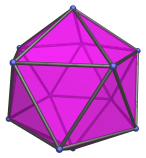It can be cut into two pentagonal pyramids and a pentagonal antiprism, or a pentagonal pyramid and a gyroelongated pentagonal pyramid (J11). It can also be cut by two non-parallel planes to produce the metabidiminished icosahedron (J62), or by 3 planes to form the tridiminished icosahedron (J63).

## Projections

In order to be able to identify the icosahedron in various projections of 4D objects, it is useful to know how it appears from various viewpoints. The following are some of the viewpoints that are commonly encountered:

Projection Envelope Description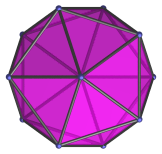Regular decagon

Vertex-first parallel projection.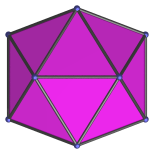Hexagon

Edge-first parallel projection. Four of the icosahedron's faces project onto the top two and bottom two edges of the projection envelope.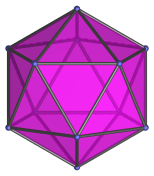Regular hexagon

Face-first parallel projection.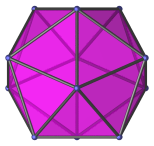Non-uniform octagon

Parallel projection, parallel to two opposite faces. The two opposite faces project to the top and bottom edges of the projection envelope.

## Coordinates

The Cartesian coordinates of an origin-centered regular icosahedron of edge length 2 are:

• (0, ±1, ±φ)
• (±1, ±φ, 0)
• (±φ, 0, ±1)

where φ=(1+√5)/2 is the Golden Ratio.

## Occurrences

The icosahedron occurs as cells in the following 4D uniform polytopes:

In addition, it also occurs in many CRF polychora, including (but not limited to):

Last updated 21 Mar 2019.